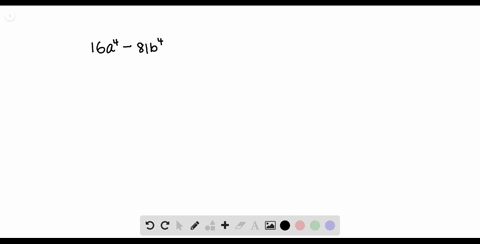Enroll in one of our FREE online STEM summer camps. Space is limited so join now!View Summer Courses### Factor each polynomial. If a polynomial cannot be…

01:51Other Schools

Need more help? Fill out this quick form to get professional live tutoring.

Get live tutoring
Problem 31

Factor each polynomial. If a polynomial cannot be factored, write prime. Factor out the greatest common factor as necessary.
$$x^{4}-y^{4}$$

$x^{4}-y^{4}=(x+y)(x-y)$

## Discussion

You must be signed in to discuss.

## Video Transcript

factor this expression here you to realize that this is actually a difference of squares that's the power for is actually exclude also very by the power for its actually y squared. Also so using difference of squares which tells us Oh yeah, minus east that gets factored into eight plus e turns a minus. So that means our expression here is excluded. Waas twice there and x squared minus y squared. So the first term, um, his partner to be kept factor that. But the second term, as we can see, is again a difference of squares to that Music can fracture that second term again so that used as becomes expect voice three because we can't factor that and an explorer minus y squared becomes exp less Why trains x minus way